# Fitting a pytorch model

Out of the box when fitting pytorch models we typically run through a manual loop. So typically something like this:

``````# Example fitting a pytorch model
# mod is the pytorch model object
crit = torch.nn.MSELoss(reduction='mean')
for t in range(20000):
y_pred = mod(x)   #x is tensor of independent vars
loss = crit(y_pred,y) #y is tensor of outcomes
loss.backward()
opt.step()``````

And this would use backpropogation to adjust our model parameters to minimize the loss function, here just the mean square error, over 20,000 iterations. Best practices are to both evaluate the loss in-sample and wait for it to flatten out, as well as evaluate out of sample.

I recently wrote some example code to make this process somewhat more like the sklearn approach, where you instantiate an initial model object, and then use a `mod.fit(X, y)` function call to fit the pytorch model. For an example use case I will just use a prior Compas recidivism data I have used for past examples on the blog (see ROC/Calibration plots, and Balancing False Positives). Here is the prepped CSV file to download to follow along.

So first, I load the libraries and then prep the recidivism data before I fit my predictive models.

``````###############################################
# Front end libraries/data prep

import pandas as pd
import numpy as np
import matplotlib.pyplot as plt
import torch

# Setting seeds
torch.manual_seed(10)
np.random.seed(10)

# Prepping the Compas data and making train/test

#Preparing the variables I want
recid_prep = recid[['Recid30','CompScore.1','CompScore.2','CompScore.3',
recid_prep['Male'] = 1*(recid['sex'] == "Male")
recid_prep['Fel'] = 1*(recid['c_charge_degree'] == "F")
recid_prep['Mis'] = 1*(recid['c_charge_degree'] == "M")
dum_race = pd.get_dummies(recid['race'])

# White for reference category
for d in list(dum_race):
if d != 'Caucasion':
recid_prep[d] = dum_race[d]

# reference category is separated/unknown/widowed
dum_mar = pd.get_dummies(recid['marital_status'])
recid_prep['Single'] = dum_mar['Single']
recid_prep['Married'] = dum_mar['Married'] + dum_mar['Significant Other']

#Now generating train and test set
recid_prep['Train'] = np.random.binomial(1,0.75,len(recid_prep))
recid_train = recid_prep[recid_prep['Train'] == 1].copy()
recid_test = recid_prep[recid_prep['Train'] == 0].copy()

#Independant variables
ind_vars = ['CompScore.1','CompScore.2','CompScore.3',
'African-American','Asian','Hispanic','Native American','Other',
'Single','Married']

# Dependent variable
y_var = 'Recid30'
###############################################``````

Now next part is more detailed, but it is the main point of the post. Typically we will make a pytorch model object something like this. Here I have various switches, such as the activation function (tanh or relu or pass in your own function), or the final function to limit predictions to 0/1 (either sigmoid or clamp or again pass in your own function).

``````# Initial pytorch model class
class logit_pytorch(torch.nn.Module):
def __init__(self, nvars, device, activate='relu', bias=True,
final='sigmoid'):
"""
Construct parameters for the coefficients
activate - either string ('relu' or 'tanh',
or pass in your own torch function
bias - whether to include bias (intercept) in model
final - use either 'sigmoid' to squash to probs, or 'clamp'
or pass in your own torch function
device - torch device to construct the tensors
default cuda:0 if available
"""
super(logit_pytorch, self).__init__()
# Creating the coefficient parameters
self.coef = torch.nn.Parameter(torch.rand((nvars,1),
device=device)/10)
# If no bias it is 0
if bias:
self.bias = torch.nn.Parameter(torch.zeros(1,
device=device))
else:
self.bias = torch.zeros(1, device=device)
# Various activation functions
if activate == 'relu':
self.trans = torch.nn.ReLU()
elif activate == 'tanh':
self.trans = torch.nn.Tanh()
else:
self.trans = activate
if final == 'sigmoid':
self.final = torch.nn.Sigmoid()
elif final == 'clamp':
# Defining my own clamp function
def tclamp(input):
self.final = tclamp
else:
# Can pass in your own function
self.final = final
def forward(self, x):
"""
predicted probability
"""
output = self.bias + torch.mm(x, self.trans(self.coef))
return self.final(output)``````

To use this though again we need to specify the number of coefficients to create, and then do a bunch of extras like the optimizer, and stepping through the function (like described at the beginning of the post). So here I have created a second class that behaves more like sklearn objects. I create the empty object, and only when I pass in data to the `.fit()` method it spins up the actual pytorch model with all its tensors of the correct dimensions.

``````# Creating a class to instantiate model to data and then fit
class pytorchLogit():
def __init__(self, loss='logit', iters=25001,
activate='relu', bias=True,
final='sigmoid', device='gpu',
printn=1000):
"""
loss - either string 'logit' or 'brier' or own pytorch function
iters - number of iterations to fit (default 25000)
activate - either string ('relu' or 'tanh',
or pass in your own torch function
bias - whether to include bias (intercept) in model
final - use either 'sigmoid' to squash to probs, or 'clamp'
or pass in your own torch function. Should not use clamp
with default logit loss
opt - ?optimizer? should add an option for this
device - torch device to construct the tensors
default cuda:0 if available
printn - how often to check the fit (default 1000 iters)
"""
super(pytorchLogit, self).__init__()
if loss == 'logit':
self.loss = torch.nn.BCELoss()
self.loss_name = 'logit'
elif loss == 'brier':
self.loss = torch.nn.MSELoss(reduction='mean')
self.loss_name = 'brier'
else:
self.loss = loss
self.loss_name = 'user defined function'
# Setting the torch device
if device == 'gpu':
try:
self.device = torch.device("cuda:0")
print(f'Torch device GPU defaults to cuda:0')
except:
print('Unsuccessful setting to GPU, defaulting to CPU')
self.device = torch.device("cpu")
elif device == 'cpu':
self.device = torch.device("cpu")
else:
self.device = device #can pass in whatever
self.iters = iters
self.mod = None
self.activate = activate
self.bias = bias
self.final = final
self.printn = printn
# Other stats to carry forward
self.loss_metrics = []
self.epoch = 0
def fit(self, X, y, outX=None, outY=None):
x_ten = torch.tensor(X.to_numpy(), dtype=torch.float,
device=self.device)
y_ten = torch.tensor(pd.DataFrame(y).to_numpy(), dtype=torch.float,
device=self.device)
# Only needed if you pass in an out of sample to check as well
if outX is not None:
x_out_ten = torch.tensor(outX.to_numpy(), dtype=torch.float,
device=self.device)
y_out_ten = torch.tensor(pd.DataFrame(outY).to_numpy(), dtype=torch.float,
device=self.device)
self.epoch += 1
# If mod is not already created, create a new one, else update prior
if self.mod is None:
loc_mod = logit_pytorch(nvars=X.shape, activate=self.activate,
bias=self.bias, final=self.final,
device=self.device)
self.mod = loc_mod
else:
loc_mod = self.mod
crit = self.loss
for t in range(self.iters):
y_pred = loc_mod(x_ten)
loss = crit(y_pred,y_ten)
if t % self.printn == 0:
if outX is not None:
pred_os = loc_mod(x_out_ten)
loss_os = crit(pred_os,y_out_ten)
res_tup = (self.epoch, t, loss.item(), loss_os.item())
print(f'{t}: insample {res_tup:.4f}, outsample {res_tup:.4f}')
else:
res_tup = (self.epoch, t, loss.item(), None)
print(f'{t}: insample {res_tup:.5f}')
self.loss_metrics.append(res_tup)
loss.backward()
opt.step()
def predict_proba(self, X):
x_ten = torch.tensor(X.to_numpy(), dtype=torch.float,
device=self.device)
res = self.mod(x_ten)
pp = res.cpu().detach().numpy()
return np.concatenate((1-pp,pp), axis=1)
def loss_stats(self, plot=True, select=0):
pd_stats = pd.DataFrame(self.loss_metrics, columns=['epoch','iteration',
'insamploss','outsamploss'])
if plot:
pd_stats2 = pd_stats.rename(columns={'insamploss':'In Sample Loss', 'outsamploss':'Out of Sample Loss'})
pd_stats2 = pd_stats2[pd_stats2['iteration'] > select].copy()
ax = pd_stats2[['iteration','In Sample Loss','Out of Sample Loss']].plot.line(x='iteration',
ylabel=f'{self.loss_name} loss')
plt.show()
return pd_stats``````

Again it allows you to pass in various extras, which here are just illustrations for binary predictions (like the loss function as the Brier score or the more typical log-loss). It also allows you to evaluate the fit for just in-sample, or for out of sample data as well. It also allows you to specify the number of iterations to fit.

So now that we have all that work done, here as some simple examples of its use.

``````# Creating a model and fitting
mod = pytorchLogit()
mod.fit(recid_train[ind_vars], recid_train[y_var])``````

So you can see that this is very similar now to sklearn functions. It will print at the console fit statistics over the iterations: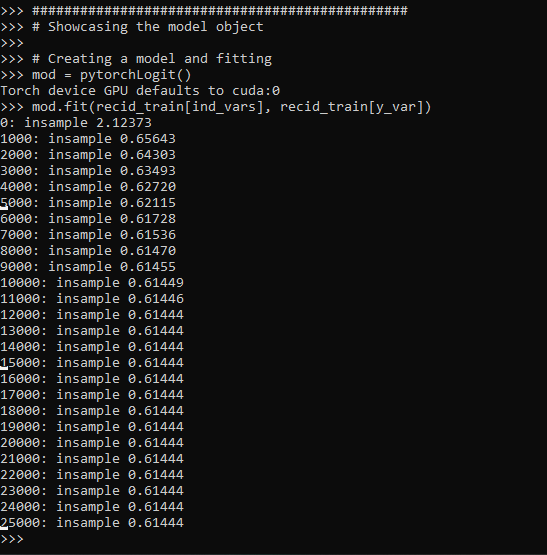So it defaults to 25k iterations, and you can see that it settles down much before that. I created a `predict_proba` function, same as most sklearn model objects for binary predictions:

``````# Predictions out of sample
predprobs = mod.predict_proba(recid_test[ind_vars])
predprobs # 1st column is probability 0, 2nd prob 1``````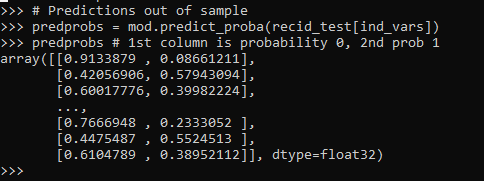And this returns a numpy array (not a pytorch tensor). Although you could modify to return a pytorch tensor if you wanted it to (or give an option to specify which).

Here is an example of evaluating out of sample fit as well, in addition to specifying a few more of the options.

``````# Evaluating predictions out of sample, more iterations
mod2 = pytorchLogit(activate='tanh', iters=40001, printn=100)
mod2.fit(recid_train[ind_vars], recid_train[y_var], recid_test[ind_vars], recid_test[y_var])``````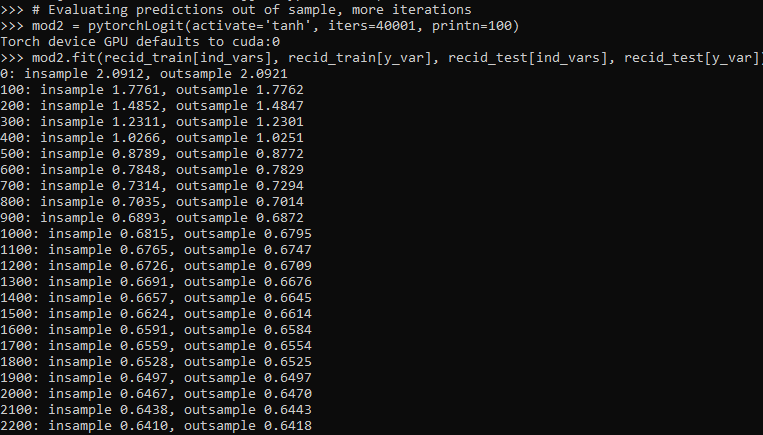I also have an object function, `.loss_stats()`, which gives a nice graph of in-sample vs out-of-sample loss metrics.

``````# Making a nice graph
dp = mod2.loss_stats()``````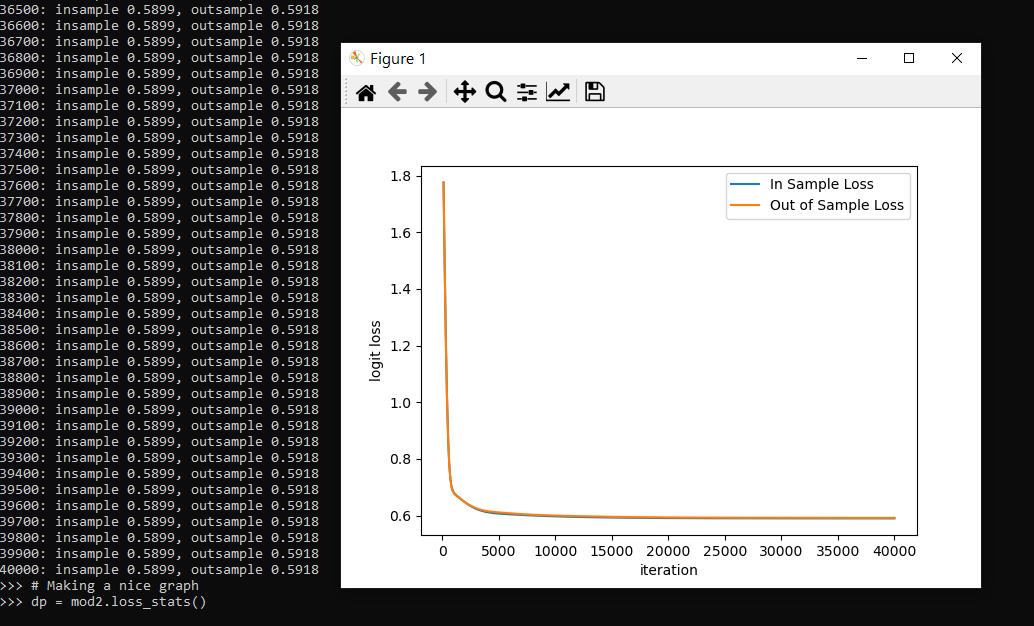We can also select the loss function to only show later iterations, so it is easier to zoom into the behavior.

``````# Checking out further along
mod2.loss_stats(select=10000)``````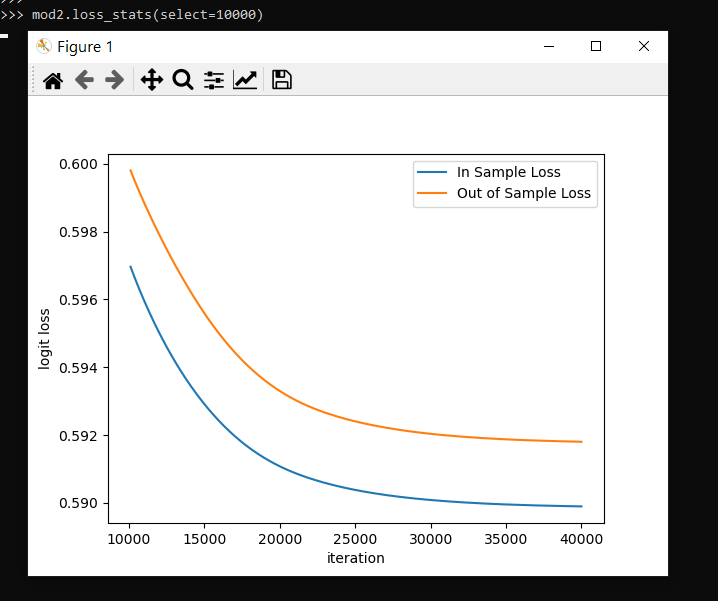And finally like I said you could modify some of your own functions here. So instead of any activation function I pass in the identity function – so this turns the model into something very similar to a vanilla logistic regression.

``````# Inserting in your own activation (here identity function)
def ident(input):
return input

mod3 = pytorchLogit(activate=ident, iters=40001, printn=2000)
mod3.fit(recid_train[ind_vars], recid_train[y_var], recid_test[ind_vars], recid_test[y_var])``````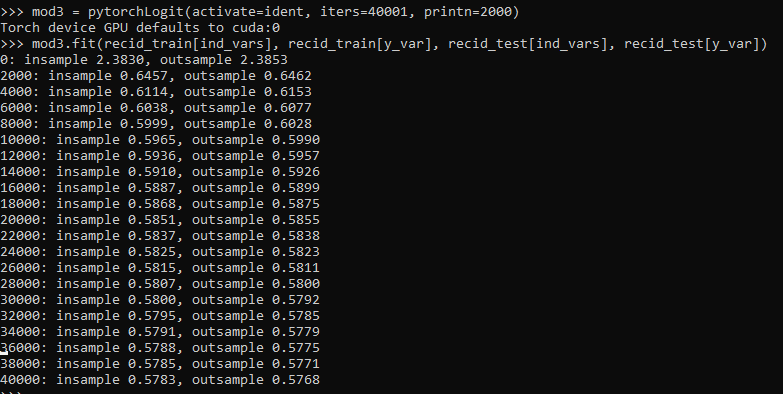And then if you want to access the coefficients weights, it is just going down the rabbit hole to the pytorch object:

``````# Can get the coefficients/intercept
print( mod3.mod.coef )
print( mod3.mod.bias )``````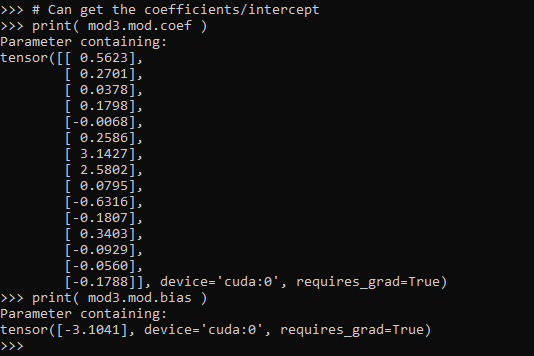This type of model can of course be extended however you want, but modifying the `pytorchLogit()` and `logit_pytorch` class objects to specify however detailed switches you want. E.g. you could specify adding in hidden layers.

One thing I am not 100% sure the best way to accomplish is loss functions that take more parameters, as well as the best way to set up the optimizer. Maybe use `*kwargs` for the loss function. So for my use cases I have stuffed extra objects into the initial class, so they are there later if I need them.

Also here I would need to think more about how to save the model to disk. The model is simple enough I could dump the tensors to numpy, and on loading re-do them as pytorch tensors.